Latest Banking jobs   »

# Reasoning Ability Quiz For IBPS Clerk Prelims 2022- 28th July

Directions (1-5): Answer the questions based on the information given below.
There are eight persons A, B, C, D, E, F, G and H who live in a building having four floors such that the ground floor is numbered as 1 and the floor immediately above the ground floor is numbered as 2 and so on up to the top floor is numbered as 4.
Each of the floors consists of two types of flats i.e., flat-A and flat -B. Flat-A of floor 2 is immediately above the flat – A of floor 1 and immediately below flat – A of floor 3 and in the same way flat -B of floor 2 is immediately above the flat – B of floor 1 and immediately below the flat-B of floor 3 and so on. Also, flat -A is in the west of flat-B. Only one person lives in each flat. Each of the flats is of the same dimension.
G lives in flat-B. H lives to the west of F. H and A live in the same type of flat. A lives above H. C and B live on the same floor. B lives below the floor of D and both live in the same type of flat. E lives above G. E and G do not live in the same type of flat. There is one floor between G and F’s floor. E lives on an even-numbered floor.

Q1. Who among the following lives in Flat A of floor 3?
(a) D
(b) A
(c) G
(d) B
(e) None of these

Q2. Who lives immediately above G in same type of flat as G lives?
(a) B
(b) C
(c) A
(d) D
(e) None of these

Q3. Who among the following lives in Flat A of floor 2?
(a) D
(b) H
(c) C
(d) None of these
(e) E

Q4. Who lives exactly between G and F in the same type of flat?
(a) D
(b) A
(c) B
(d) Either E or D
(e) E

Q5. Which of the following statement is true?
(a) D lives below F in the same type of flat
(b) A lives above D
(c) E and H live on consecutive floors
(d) D lives west of E
(e) B and F live in same type of flat

Directions (6-10): Answer the questions based on the information given below.
Ten boxes M, N, O, P, Q, R, S, T, U, and V are kept above one another such that the bottommost box is 1 and the box above it is 2 and so on. Three boxes are kept between Box V and Box S. Box Q is kept just above Box U and both the boxes are kept above Box V. Four boxes are kept between Box Q and Box N. Three boxes are kept between Box T and Box R which is not kept above Box N. Three boxes are kept between Box M and Box O. At most 2 boxes are kept below O. The number of boxes kept below Box O is same as the number of boxes kept above Box V.

Q6. Which of the following statements is/are true?
(a) Two boxes are kept between box U and box T
(b) Box M is kept just above Box N
(c) Box R is bottommost box
(d) Box U is topmost box
(e) None of the above

Q7. Which box is kept just above box P?
(a) O
(b) R
(c) M
(d) S
(e) Either O or M

Q8. How many boxes are kept between Box M and Box P?
(a) One
(b) Two
(c) Three
(d) Four
(e) Five

Q9. Which of the following box is the bottommost box?
(a) R
(b) T
(c) P
(d) S
(e) None of these

Q10. Four of the following five are alike in a certain way and hence form a group. Which is the one that doesn’t belong to that group?
(a) Box Q and V
(b) Box V and T
(c) Box T and S
(d) Box S and R
(e) Box O and R

Directions (11-13): In the following questions assuming the given statement to be true, find which of the conclusion(s) among given conclusions is/are definitely true and then give your answers accordingly.

Q11. Statement: X = P ≥ Q = R > S, S < T ≤ U = V < W
Conclusions: I. X > S
II. R < W
(a) Only I is true
(b) Only II is true
(c) Either I or II is true
(d) Neither I nor II is true
(e) Both I and II are true

Q12. Statement: C = A ≥ D > B, B < E ≥ F > G > H
Conclusions: I. C > G
II. H < E
(a) Only I is true
(b) Only II is true
(c) Either I or II is true
(d) Neither I nor II is true
(e) Both I and II are true

Q13. Statement: I < J ≤ K, K = L ≥ M, M > N ≥ O > P
Conclusions: I. P < K
II. L > O
(a) Only I is true
(b) Only II is true
(c) Either I or II is true
(d) Neither I nor II is true
(e) Both I and II are true

Q14. Which of the following symbols will replace the blanks in the given expression in order to make the expression U < V & X > Y definitely true?
Z > V ≥ W _____ Y = U _____ X
(a) =, <
(b) =, >
(c) <, >
(d) >, <
(e) None of the above

Q15. Which of the following symbols will replace the blanks in the given expression in order to make the expression E ≥ H & F > J definitely true?
D > E = F ≥ G _____ H > I _____ J
(a) >, =
(b) <, =
(c) ≥, ≥
(d) ≤, ≤
(e) None of the above

Solutions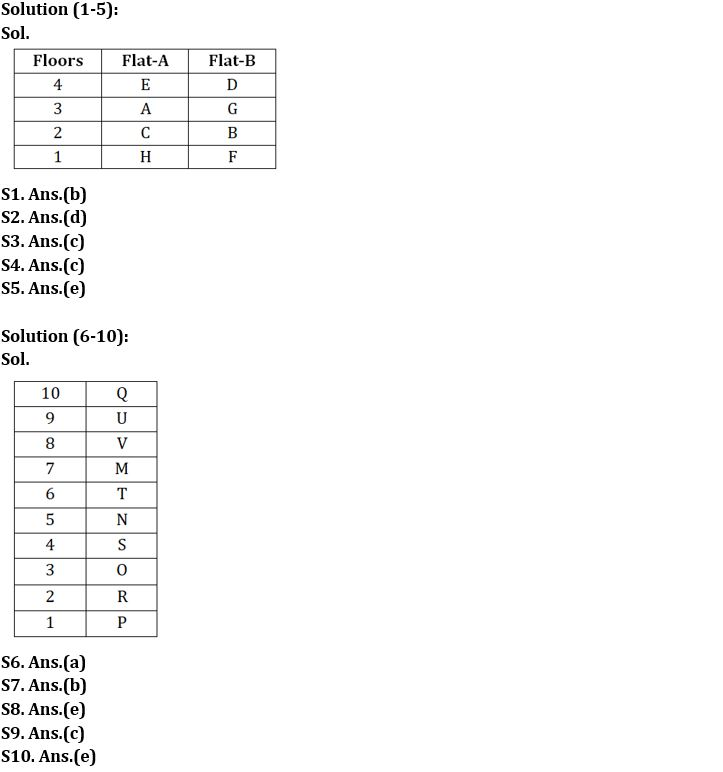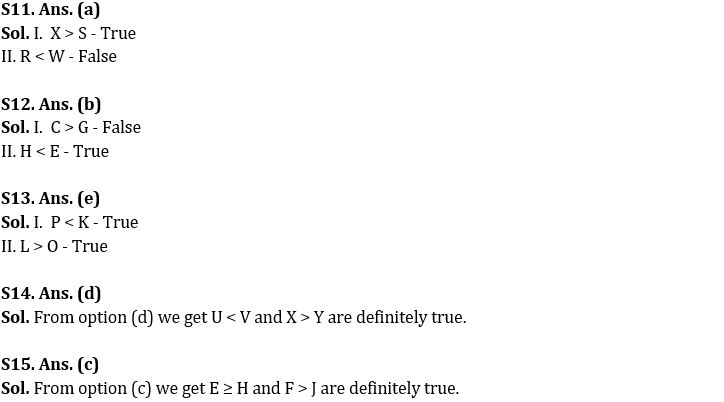#### Congratulations!Incorrect details? Fill the form again here

•Reasoning Ability Quiz For IBPS RRB PO C...
•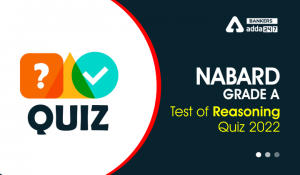Reasoning Ability Quiz For NABARD Grade ...
•Reasoning Ability Quiz For IBPS RRB PO C...
•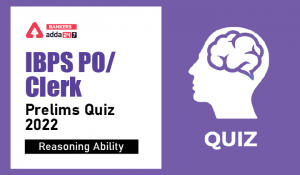Reasoning Ability Quiz For IBPS Clerk/PO...
•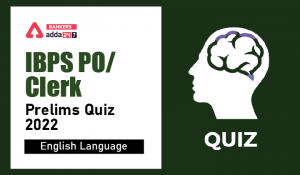English Quizzes For IBPS Clerk/PO Prelim...
•English Quizzes For IBPS Clerk/PO Prelim...